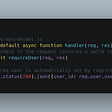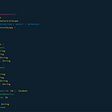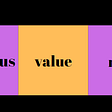# Data Structure: Selection Sort

What is the Selection sort?

The selection sort method is a useful method. This method will take an array of unsorted numbers and sort them from small to large depending on your condition. The way it is able to perform this method is by finding the minimum element Index.

How does it work

Since we are storing the indices of each element we need to create a variable.

`let min;`

Once the variable is established we need to iterate through the array.

`for(let i = 0; i < array.length - 1; i++){`

Notice how the loop is formed with array.length — 1. This is because once we sort a set of arrays we will decrease the number of elements in that array. Upon setting up the first set of iteration, the variable min needs a value. We can set min to I since the value is zero.

`for(let i = 0; i < array.length - 1; i++){        min = i`

The second iteration starts at the first index.

`for(let j = i + 1; j < array.length; j++){`

. Furthermore inside of the loop, a conditional is needed to be added. If the first index is less than the one before. We set the variable to the index.

`if(array[j] < array[min]) min = j`

Each value that has the least value compared to the index before it will be stored by index.

Just to make sure our loop goes as planned. We break out of our loop to add another conditional that stops the current index and variable to equal to another.

`if(i !== min){`

If the condition evaluates to true we will proceed to swap the two values and return the array in the correct order.

`if(i !== min){        let temp = array[i]        array[i] = array[min]        array[min] = temp    }`

Here’s a full view of the algorithm.

`function selectionSort(array) {    let min;for(let i = 0; i < array.length - 1; i++){        min = ifor(let j = i + 1; j < array.length; j++){            if(array[j] < array[min]) min = j        }if(i !== min){        let temp = array[i]        array[i] = array[min]        array[min] = temp    }    }return array}`

The time complexity is considered to be O(n2). Looping twice through an array to return our ordered elements. Leave a comment below for any question

--

--

--

## More from Woodelin florveus

Love podcasts or audiobooks? Learn on the go with our new app.

## Fastify and Sequelize with TypeScript.## How to mint on RMRK for the Zeitgeist Seer program NFT Artist Showcase## Why Opt for AngularJS?## Fast Serverless Authentication with Next.js and PropelAuth## Start GraphQL on ApolloServer With Express JS Within 5 minutes## YouTube Video | YDKJS — Scopes And Closures — 5## Creating Stellar Animations in React Native Using Reanimated 2.0## Integrate Salesforce and Sharepoint using Aura component## More from Medium## A SHED UNDER THE TREE DATA STRUCTURE## Two Sorting Algorithms You Must Know About — Quick Sort and Merge Sort## Simulating Flocking Behavior In Virtual Agents